Engineering Math - Quick Reference                                 Home : www.sharetechnote.com Matrix-LU Decomposition   LU decomposition is a method to split (decompose) a matrix into two matrix product. One of these two matrix(the first part) is called 'L' matrix meaning 'Lower diagonal matrix' and the other matrix(the second part) is called 'U' matrix meaning 'Upper diagonal matrix'. Simply put, LU decomposition is a process to convert a Matrix A into the product of L and U as shown below.   A = LU   I would not explain about how you can decompose a matrix into LU form. The answer is "Use software" -:). I would talk about WHY.   Let's assume that we have a matrix equation (Linear Equation) as shown below.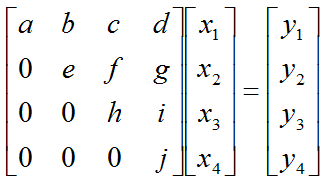Don't look into each elements yet. Just get around 100 steps back away from this and look at the overall pattern of the matrix. Can you see a pattern as shown below ? You see two area marked as triangles. Green triangle shows all the elements from diagonal line and upper diagonal part. The elements in this triangle is non-zero values. Violet triangle shows the elements of lower diagonal part which is all zero.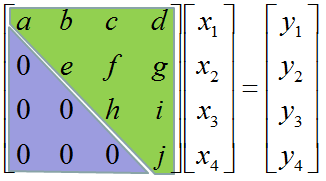This form of matrix is called 'U' form matrix, meaning 'Upper diagonal matrix'. Why this is so special ? It is special because it is so easy to solve the equation.  Now let's think about how we can solve this equation. Let's look the last row (4th row in this case). How can I figure out the value for x4 ? You would get it right away because there is only x4 term and j and y4 are known values. Once you get x4 and plug in the x4 value into row 3, then you would get x3 value. Once you get x3 and plug in the x3 value into row 2, then you would get x2 value. Once you get x2 and plug in the x2 value into row 1, then you would get x1 value.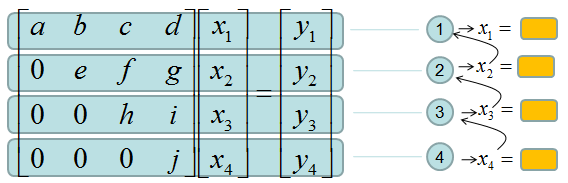If it is not clear with you, it would be a little clearer if you convert the matrix equation into simultaneous equation as shown below.   Start from the last equation and get x4 first and repeat the process described above.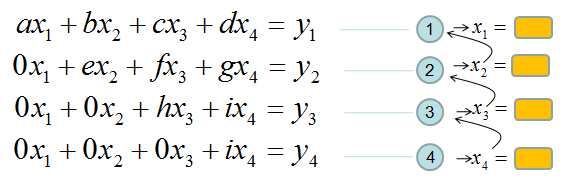If this process is still unclear,just play with real numbers. Put any numbers for a,b,c,d,e,f,g,h,i,y1,y2,y3,y4 and try to get x1,x2,x3,x4 as explained above.   If you tried this as explained, one thing you would notice would be "It is so simple to find the solution x1,x2,x3,x4". Just compare this process with what you experienced with general matrix equation solving process you did in your high school math or linear algebra class.   Now let's look into another example of matrix equation as shown below.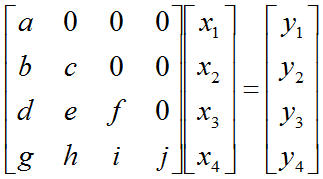Now you would know what I will say. In this case, all the elements along the diagonal line and Lower part of diagonal line is non-zero values. All the values above the diagonal line is all zero. This form is called 'L' form meaning 'Lower diagonal matrix'.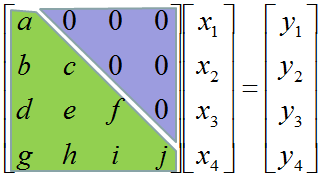Why this is so special ? It is special because it is so easy to solve the equation.  Now let's think about how we can solve this equation. Let's look the first row (the first row in this case). How can I figure out the value for x1 ? You would get it right away because there is only x1 term and a and y1 are known values. Once you get x1 and plug in the x1 value into row 2, then you would get x2 value. Once you get x2 and plug in the x3 value into row 3, then you would get x3 value. Once you get x3 and plug in the x2 value into row 4, then you would get x4 value.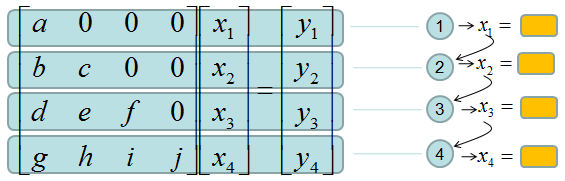If it is not clear with you, it would be a little clearer if you convert the matrix equation into simultaneous equation as shown below.   Start from the first equation and get x1 first and repeat the process described above.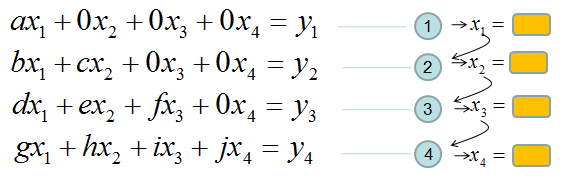If this process is still unclear,just play with real numbers. Put any numbers for a,b,c,d,e,f,g,h,i,y1,y2,y3,y4 and try to get x1,x2,x3,x4 as explained above.   If you tried this as explained, one thing you would notice would be "It is so simple to find the solution x1,x2,x3,x4". Just compare this process with what you experienced with general matrix equation solving process you did in your high school math or linear algebra class.   Now let's suppose we have a matrix as shown below. Here all the elements in the matrix is non-zero values.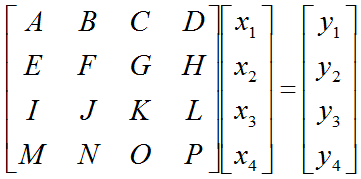Now let's assume we can convert this matrix equation into following form. (Don't worry about HOW, just assume you can do this somehow). You already know that this form would make it easier to solve the equation.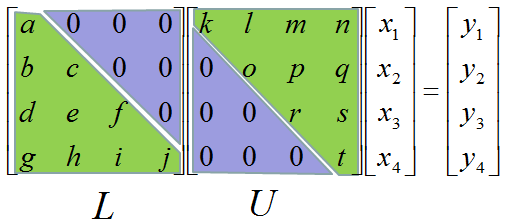Try this example that I found from web. (If the link is missing, try here).   Now you may say.. "You always say 'don't worry about how to solve. just use computer software'". If I use the computer software, why do I have to worry about this kind of conversion. Computer software would not have any problem to solve the equation even with the original form without LU decomposition. You are right, the computer program would not have any problem with original form.But the amount of time for the calculation is much shorter with LU decomposed form than the original form. When the size of matrix, this time difference would be negligiable, but if the size is very huge (e.g, 10000 x 10000) the time difference would be very huge. If you've done a computer science, you would be familiar with Big O notation to evaluate the computation time. Try compare the computation time for the original matrix form and LU decomposed form. If you are not familiar with this notation, just trust me -:). Following two YouTube tutorial would give you some insight of LU decomposition including computation time.